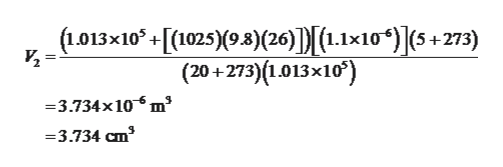At 26.0 m below the surface of the sea (density = 1 025 kg/m3), where the temperature is 5.00°C, a diver exhales an air bubble having a volume of 1.10 cm3. If the surface temperature of the sea is 20.0°C, what is the volume of the bubble just before it breaks the surface in centimeters?

Question

At 26.0 m below the surface of the sea (density = 1 025 kg/m3), where the temperature is 5.00°C, a diver exhales an air bubble having a volume of 1.10 cm3. If the surface temperature of the sea is 20.0°C, what is the volume of the bubble just before it breaks the surface in centimeters?

Step 1

The expression for the final volume from ideal gas law,

Step 2

Substitute the ...help_outlineImage Transcriptionclose(1.013x10 +[(1025)(9.3)(26)]) [L.1x10*)|(5 + 273) (20+273)(1.013x10) 3.734x10 m' -3.734 cm3 fullscreen

Want to see the full answer?

See Solution

Want to see this answer and more?

Our solutions are written by experts, many with advanced degrees, and available 24/7

See Solution
Tagged in

Physics